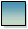### The number of hours left in a day is half the number of hours already passed. How many hours are left in the day?

8 hours

Step by Step Explanation:
1. According to the question,
Number of hours left in the day =
 1 2
× Number of hours already passed
2. Let the number of hours already passed be.
3. So, the number of hours left in the day =2

4. We know that the total number of hours in a day = 24
So,+2
= 24
or,
 32
= 24
or,=
 24 × 2 3
= 16
5. Therefore, the number of hours left in the day =
 16 2
= 8 hours